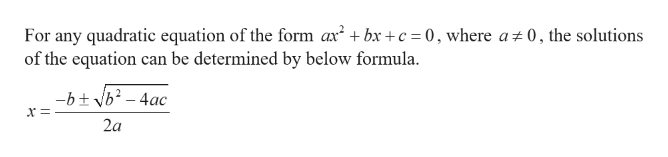# I need help understanding how to solve eqations using the quadratic formula.

Question
6 views

I need help understanding how to solve eqations using the quadratic formula.

check_circle

star
star
star
star
star
1 Rating
Step 1help_outlineImage TranscriptioncloseFor any quadratic equation of the form ax* + bx + c = 0, where a0, the solutions of the equation can be determined by below formula. -btb2-4ac 2a fullscreen
Step 2

Let us consider an equation.

Step 3

Compare the above equation with standard quadratic equation.

We have a = 2, b = 7 and c = 1.

The...

### Want to see the full answer?

See Solution

#### Want to see this answer and more?

Solutions are written by subject experts who are available 24/7. Questions are typically answered within 1 hour.*

See Solution
*Response times may vary by subject and question.
Tagged in

### Algebra